## June 16, 2007

### Rounded Values and the Quantity Column

Many times you may have objects and associated data that you would like to document in a Schedule Table, but there are large numbers of "identical" objects - all the data in each objects row is the same - and you really do not need to show each line. This is just the situation that the quantity column was meant to handle - check the box on the Columns tab when creating or editing your Schedule Table Style to add a Quantity column, as shown in the image below.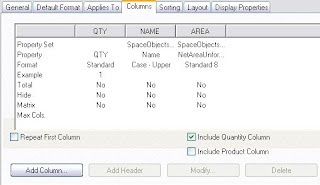With a quantity column in your schedule table, all identical rows will "collapse" into a single row, with the total number of objects represented listed in the quantity column. If you do not want to display the actual quantity, simply hide the quantity column.

An interesting question came up in this thread in the Autodesk Architectural Desktop 2007 & Prior Discussion Group. The images in this blog post were taken from the ADT 2006 drawing file I posted in reply. The goal was to list spaces and their areas in a schedule table, using a quantity column to collapse spaces of the same type and area onto one line. Unfortunately, there were slight differences in the actual areas of the spaces, such as those in my sample file, as illustrated by the schedule table below. I created an "unformatted" automatic property to which I assigned a Property Data Format that is a copy of the Standard Property Data Format, with the precision set to eight decimal places.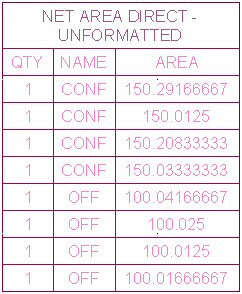There are two different types of spaces: offices, at a nominal 100 square feet, and conference rooms, at a nominal 150 square feet. Intentional (in my case) stretching of the spaces by varying small amounts resulted in the slightly different areas shown, and preventing any lines from collapsing. Given the obviously different values, this is not surprising.

The discussion group poster noted that he had created a Property Data Format for his area properties that rounded the values to the nearest whole number, but this still was not allowing the rows to collapse, despite the apparently identical values. As shown below, I was able to reproduce that in my sample file. ADT is using the raw automatic property value, before the application of the Property Data Format, to determine whether rows are identical, as can be seen in the schedule table shown below.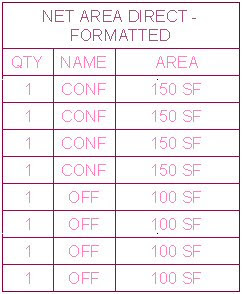Fortunately, there is a way to achieve the desired result, other than insisting on precise drafting. And while that is something of a pet peeve of mine, there certainly could be situations where there are legitimate small variations in a given value, that disappear when rounded to the desired accuracy for reporting the value. I can think of two ways to achieve the desired result, both of which involve the use of a formula property. Which works best for you will depend upon the specifics of your situation.

The first formula uses the unformatted automatic property and applies the Round function to get the desired precision, as seen below. For whole number precision, the Fix function may also work, although that will simply truncate any fractional amount, rather than round it.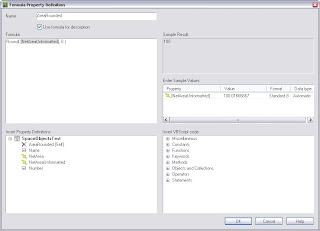The advantage of using an unformatted property to get a numeric value, then applying the Round function in a formula property is that you can control the desired precision, and, with a little math, can even get a precision greater than whole number. For example, if you wanted to have the area value rounded to the nearest 50 square feet, you could do something like this:
Round( [NetAreaUnformatted]/50, 0 ) * 50
By dividing the value by 50, rounding, then multiplying the value by 50, you will get the value, rounded to the nearest 50. One thing to note for the Round function is that if the value of the digit beyond the one being rounded is exactly 5, the digit being rounded will round up if the digit is odd and down if the digit is even (that is, the result will always be an even digit). The results of using that formula in the schedule table can be seen below - the values for all four offices and all four conference rooms are now the same, so they collapse onto two lines, one for each type.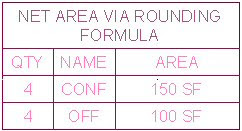The second formula assumes that you can achieve the desired rounding with a Property Data Format, and simply takes the formatted version of the automatic property and passes it through the formula, enclosed in double quotation marks, to convert it to a string value, like this:
"[NetArea]"
Since the Property Data Format made the displayed values for each space type the same, the string will be the same, and the rows will collapse, as seen in the image below.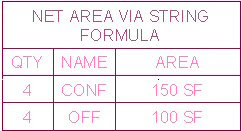If you are interested, you can download the sample file I posted to the thread and take a look at the formulas "live".